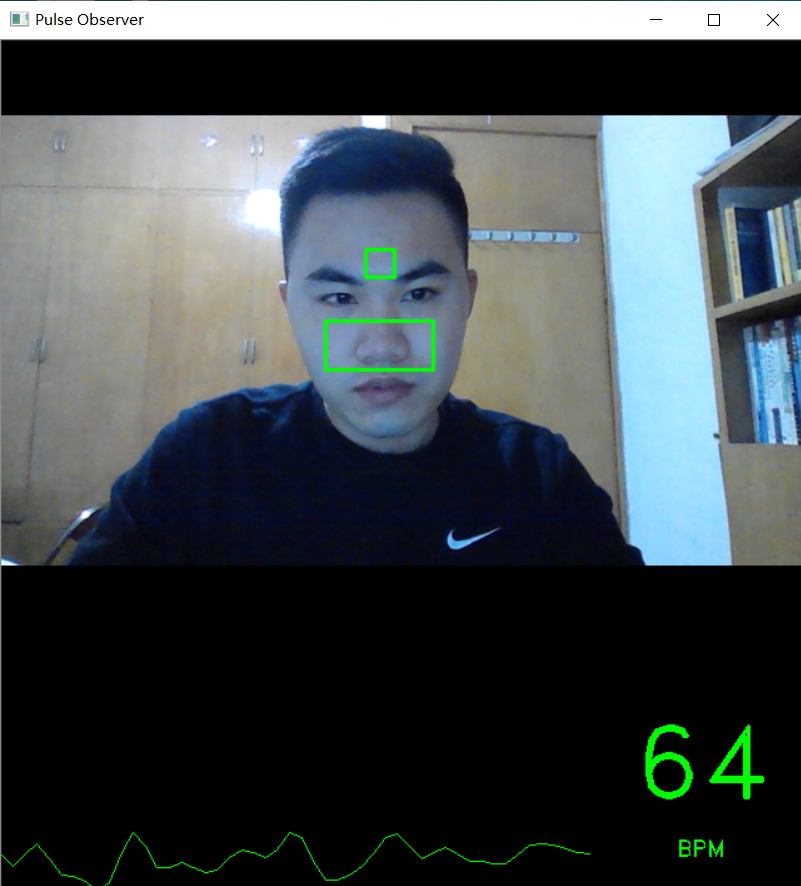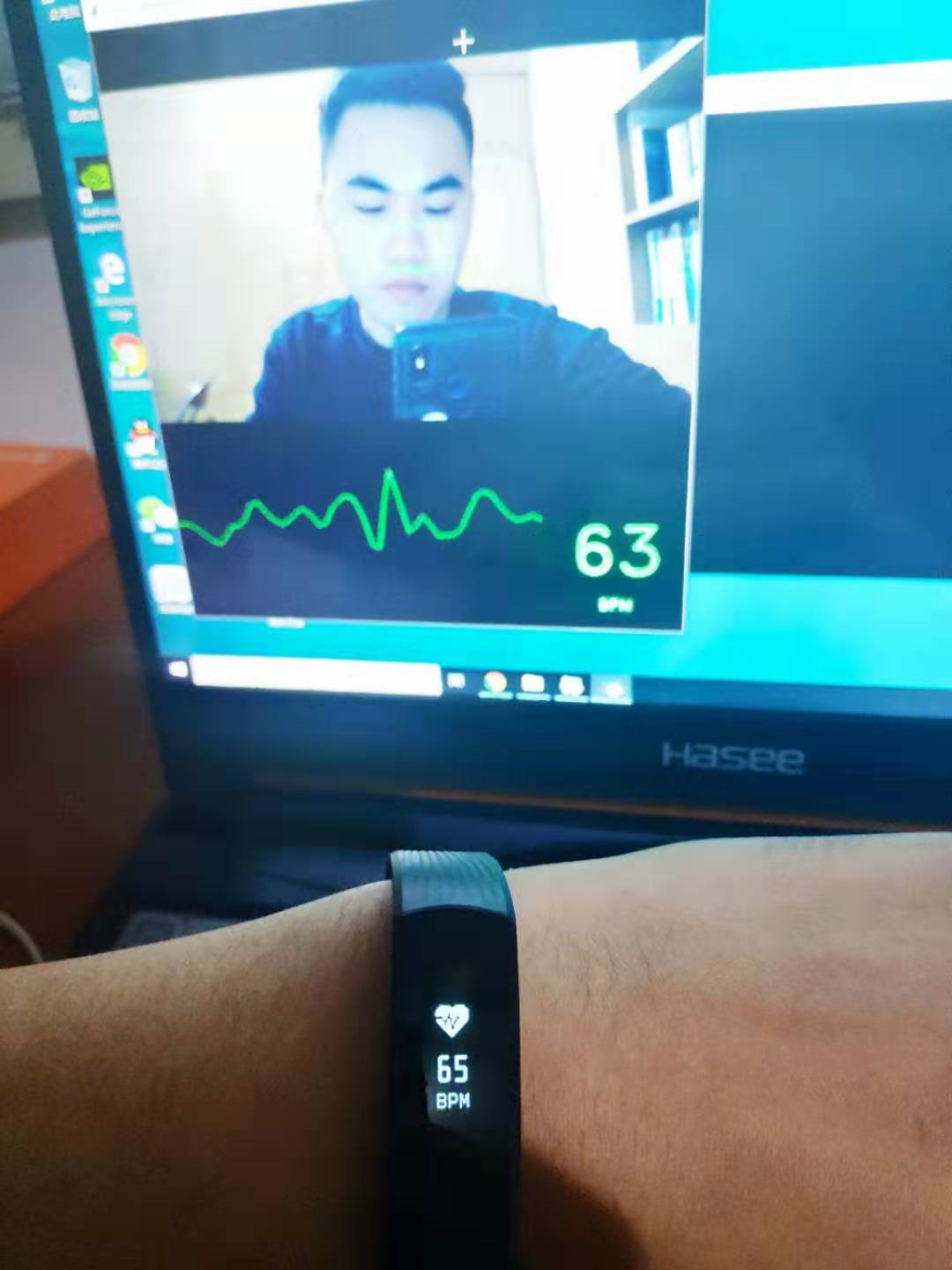# Python+OpenCV使用摄像头追踪人脸面部血液变化实现脉搏评估import cv2
import numpy as np
import dlib
import time
from scipy import signal

# Constants
WINDOW_TITLE = 'Pulse Observer'
BUFFER_MAX_SIZE = 500       # Number of recent ROI average values to store
MAX_VALUES_TO_GRAPH = 50    # Number of recent ROI average values to show in the pulse graph
MIN_HZ = 0.83       # 50 BPM - minimum allowed heart rate
MAX_HZ = 3.33       # 200 BPM - maximum allowed heart rate
MIN_FRAMES = 100    # Minimum number of frames required before heart rate is computed.  Higher values are slower, but
# more accurate.
DEBUG_MODE = False

# Creates the specified Butterworth filter and applies it.
def butterworth_filter(data, low, high, sample_rate, order=5):
nyquist_rate = sample_rate * 0.5
low /= nyquist_rate
high /= nyquist_rate
b, a = signal.butter(order, [low, high], btype='band')
return signal.lfilter(b, a, data)

# Gets the region of interest for the forehead.
# Store the points in a Numpy array so we can easily get the min and max for x and y via slicing
points = np.zeros((len(face_points.parts()), 2))
for i, part in enumerate(face_points.parts()):
points[i] = (part.x, part.y)

min_x = int(points[21, 0])
min_y = int(min(points[21, 1], points[22, 1]))
max_x = int(points[22, 0])
max_y = int(max(points[21, 1], points[22, 1]))
left = min_x
right = max_x
top = min_y - (max_x - min_x)
bottom = max_y * 0.98
return int(left), int(right), int(top), int(bottom)

# Gets the region of interest for the nose.
def get_nose_roi(face_points):
points = np.zeros((len(face_points.parts()), 2))
for i, part in enumerate(face_points.parts()):
points[i] = (part.x, part.y)

# Nose and cheeks
min_x = int(points[36, 0])
min_y = int(points[28, 1])
max_x = int(points[45, 0])
max_y = int(points[33, 1])
left = min_x
right = max_x
top = min_y + (min_y * 0.02)
bottom = max_y + (max_y * 0.02)
return int(left), int(right), int(top), int(bottom)

# Gets region of interest that includes forehead, eyes, and nose.
# Note:  Combination of forehead and nose performs better.  This is probably because this ROI includes eyes,
def get_full_roi(face_points):
points = np.zeros((len(face_points.parts()), 2))
for i, part in enumerate(face_points.parts()):
points[i] = (part.x, part.y)

# Only keep the points that correspond to the internal features of the face (e.g. mouth, nose, eyes, brows).
# The points outlining the jaw are discarded.
min_x = int(np.min(points[17:47, 0]))
min_y = int(np.min(points[17:47, 1]))
max_x = int(np.max(points[17:47, 0]))
max_y = int(np.max(points[17:47, 1]))

center_x = min_x + (max_x - min_x) / 2
left = min_x + int((center_x - min_x) * 0.15)
right = max_x - int((max_x - center_x) * 0.15)
top = int(min_y * 0.88)
bottom = max_y
return int(left), int(right), int(top), int(bottom)

def sliding_window_demean(signal_values, num_windows):
window_size = int(round(len(signal_values) / num_windows))
demeaned = np.zeros(signal_values.shape)
for i in range(0, len(signal_values), window_size):
if i + window_size > len(signal_values):
window_size = len(signal_values) - i
curr_slice = signal_values[i: i + window_size]
if DEBUG_MODE and curr_slice.size == 0:
print ('Empty Slice: size={0}, i={1}, window_size={2}'.format(signal_values.size, i, window_size))
print (curr_slice)
demeaned[i:i + window_size] = curr_slice - np.mean(curr_slice)
return demeaned

# Averages the green values for two arrays of pixels
def get_avg(roi1, roi2):
roi1_green = roi1[:, :, 1]
roi2_green = roi2[:, :, 1]
avg = (np.mean(roi1_green) + np.mean(roi2_green)) / 2.0
return avg

# Returns maximum absolute value from a list
def get_max_abs(lst):
return max(max(lst), -min(lst))

# Draws the heart rate graph in the GUI window.
def draw_graph(signal_values, graph_width, graph_height):
graph = np.zeros((graph_height, graph_width, 3), np.uint8)
scale_factor_x = float(graph_width) / MAX_VALUES_TO_GRAPH

# Automatically rescale vertically based on the value with largest absolute value
max_abs = get_max_abs(signal_values)
scale_factor_y = (float(graph_height) / 2.0) / max_abs

midpoint_y = graph_height / 2
for i in range(0, len(signal_values) - 1):
curr_x = int(i * scale_factor_x)
curr_y = int(midpoint_y + signal_values[i] * scale_factor_y)
next_x = int((i + 1) * scale_factor_x)
next_y = int(midpoint_y + signal_values[i + 1] * scale_factor_y)
cv2.line(graph, (curr_x, curr_y), (next_x, next_y), color=(0, 255, 0), thickness=1)
return graph

# Draws the heart rate text (BPM) in the GUI window.
def draw_bpm(bpm_str, bpm_width, bpm_height):
bpm_display = np.zeros((bpm_height, bpm_width, 3), np.uint8)
bpm_text_size, bpm_text_base = cv2.getTextSize(bpm_str, fontFace=cv2.FONT_HERSHEY_DUPLEX, fontScale=2.7,
thickness=2)
bpm_text_x = int((bpm_width - bpm_text_size) / 2)
bpm_text_y = int(bpm_height / 2 + bpm_text_base)
cv2.putText(bpm_display, bpm_str, (bpm_text_x, bpm_text_y), fontFace=cv2.FONT_HERSHEY_DUPLEX,
fontScale=2.7, color=(0, 255, 0), thickness=2)
bpm_label_size, bpm_label_base = cv2.getTextSize('BPM', fontFace=cv2.FONT_HERSHEY_DUPLEX, fontScale=0.6,
thickness=1)
bpm_label_x = int((bpm_width - bpm_label_size) / 2)
bpm_label_y = int(bpm_height - bpm_label_size * 2)
cv2.putText(bpm_display, 'BPM', (bpm_label_x, bpm_label_y),
fontFace=cv2.FONT_HERSHEY_DUPLEX, fontScale=0.6, color=(0, 255, 0), thickness=1)
return bpm_display

# Draws the current frames per second in the GUI window.
def draw_fps(frame, fps):
cv2.rectangle(frame, (0, 0), (100, 30), color=(0, 0, 0), thickness=-1)
cv2.putText(frame, 'FPS: ' + str(round(fps, 2)), (5, 20), fontFace=cv2.FONT_HERSHEY_PLAIN,
fontScale=1, color=(0, 255, 0))
return frame

# Draw text in the graph area
def draw_graph_text(text, color, graph_width, graph_height):
graph = np.zeros((graph_height, graph_width, 3), np.uint8)
text_size, text_base = cv2.getTextSize(text, fontFace=cv2.FONT_HERSHEY_DUPLEX, fontScale=1, thickness=1)
text_x = int((graph_width - text_size) / 2)
text_y = int((graph_height / 2 + text_base))
cv2.putText(graph, text, (text_x, text_y), fontFace=cv2.FONT_HERSHEY_DUPLEX, fontScale=1, color=color,
thickness=1)
return graph

# Calculate the pulse in beats per minute (BPM)
def compute_bpm(filtered_values, fps, buffer_size, last_bpm):
# Compute FFT
fft = np.abs(np.fft.rfft(filtered_values))

# Generate list of frequencies that correspond to the FFT values
freqs = fps / buffer_size * np.arange(buffer_size / 2 + 1)

# Filter out any peaks in the FFT that are not within our range of [MIN_HZ, MAX_HZ]
# because they correspond to impossible BPM values.
while True:
max_idx = fft.argmax()
bps = freqs[max_idx]
if bps < MIN_HZ or bps > MAX_HZ:
if DEBUG_MODE:
print ('BPM of {0} was discarded.'.format(bps * 60.0))
fft[max_idx] = 0
else:
bpm = bps * 60.0
break

# It's impossible for the heart rate to change more than 10% between samples,
# so use a weighted average to smooth the BPM with the last BPM.
if last_bpm > 0:
bpm = (last_bpm * 0.9) + (bpm * 0.1)

return bpm

def filter_signal_data(values, fps):
# Ensure that array doesn't have infinite or NaN values
values = np.array(values)
np.nan_to_num(values, copy=False)

# Smooth the signal by detrending and demeaning
detrended = signal.detrend(values, type='linear')
demeaned = sliding_window_demean(detrended, 15)
# Filter signal with Butterworth bandpass filter
filtered = butterworth_filter(demeaned, MIN_HZ, MAX_HZ, fps, order=5)
return filtered

# Get the average value for the regions of interest.  Will also draw a green rectangle around
# the regions of interest, if requested.
def get_roi_avg(frame, view, face_points, draw_rect=True):
# Get the regions of interest.
fh_left, fh_right, fh_top, fh_bottom = get_forehead_roi(face_points)
nose_left, nose_right, nose_top, nose_bottom = get_nose_roi(face_points)

# Draw green rectangles around our regions of interest (ROI)
if draw_rect:
cv2.rectangle(view, (fh_left, fh_top), (fh_right, fh_bottom), color=(0, 255, 0), thickness=2)
cv2.rectangle(view, (nose_left, nose_top), (nose_right, nose_bottom), color=(0, 255, 0), thickness=2)

# Slice out the regions of interest (ROI) and average them
fh_roi = frame[fh_top:fh_bottom, fh_left:fh_right]
nose_roi = frame[nose_top:nose_bottom, nose_left:nose_right]
return get_avg(fh_roi, nose_roi)

# Main function.
def run_pulse_observer(detector, predictor, webcam, window):
roi_avg_values = []
graph_values = []
times = []
last_bpm = 0
graph_height = 200
graph_width = 0
bpm_display_width = 0

# cv2.getWindowProperty() returns -1 when window is closed by user.
while cv2.getWindowProperty(window, 0) == 0:

# ret_val == False if unable to read from webcam
if not ret_val:
print ("ERROR:  Unable to read from webcam.  Was the webcam disconnected?  Exiting.")
shut_down(webcam)

# Make copy of frame before we draw on it.  We'll display the copy in the GUI.
# The original frame will be used to compute heart rate.
view = np.array(frame)

# Heart rate graph gets 75% of window width.  BPM gets 25%.
if graph_width == 0:
graph_width = int(view.shape * 0.75)
if DEBUG_MODE:
print ('Graph width = {0}'.format(graph_width))
if bpm_display_width == 0:
bpm_display_width = view.shape - graph_width

# Detect face using dlib
faces = detector(frame, 0)
if len(faces) == 1:
face_points = predictor(frame, faces)
roi_avg = get_roi_avg(frame, view, face_points, draw_rect=True)
roi_avg_values.append(roi_avg)
times.append(time.time())

# Buffer is full, so pop the value off the top to get rid of it
if len(times) > BUFFER_MAX_SIZE:
roi_avg_values.pop(0)
times.pop(0)

curr_buffer_size = len(times)

# Don't try to compute pulse until we have at least the min. number of frames
if curr_buffer_size > MIN_FRAMES:
# Compute relevant times
time_elapsed = times[-1] - times
fps = curr_buffer_size / time_elapsed  # frames per second
# Clean up the signal data
filtered = filter_signal_data(roi_avg_values, fps)

graph_values.append(filtered[-1])
if len(graph_values) > MAX_VALUES_TO_GRAPH:
graph_values.pop(0)

# Draw the pulse graph
graph = draw_graph(graph_values, graph_width, graph_height)
# Compute and display the BPM
bpm = compute_bpm(filtered, fps, curr_buffer_size, last_bpm)
bpm_display = draw_bpm(str(int(round(bpm))), bpm_display_width, graph_height)
last_bpm = bpm
# Display the FPS
if DEBUG_MODE:
view = draw_fps(view, fps)

else:
# If there's not enough data to compute HR, show an empty graph with loading text and
# the BPM placeholder
pct = int(round(float(curr_buffer_size) / MIN_FRAMES * 100.0))
bpm_display = draw_bpm('--', bpm_display_width, graph_height)

else:
# No faces detected, so we must clear the lists of values and timestamps.  Otherwise there will be a gap
# in timestamps when a face is detected again.
del roi_avg_values[:]
del times[:]
graph = draw_graph_text('No face detected', (0, 0, 255), graph_width, graph_height)
bpm_display = draw_bpm('--', bpm_display_width, graph_height)

graph = np.hstack((graph, bpm_display))
view = np.vstack((view, graph))

cv2.imshow(window, view)

key = cv2.waitKey(1)
# Exit if user presses the escape key
if key == 27:
shut_down(webcam)

# Clean up
def shut_down(webcam):
webcam.release()
cv2.destroyAllWindows()
exit(0)

def main():
detector = dlib.get_frontal_face_detector()
# http://sourceforge.net/projects/dclib/files/dlib/v18.10/shape_predictor_68_face_landmarks.dat.bz2

try:
predictor = dlib.shape_predictor('shape_predictor_68_face_landmarks.dat')
except RuntimeError as e:
return

webcam = cv2.VideoCapture(0)
if not webcam.isOpened():
print ('ERROR:  Unable to open webcam.  Verify that webcam is connected and try again.  Exiting.')
webcam.release()
return

cv2.namedWindow(WINDOW_TITLE)
run_pulse_observer(detector, predictor, webcam, WINDOW_TITLE)

# run_pulse_observer() returns when the user has closed the window.  Time to shut down.
shut_down(webcam)

if __name__ == '__main__':
main()

作者：华为云享开发者 不脱发的程序猿08-05980

#### 数据库实践丨MySQL多表join分析

05-068664

#### 【华为云技术分享】物联网常用开发板

01-211万+

#### 华为IoT首席架构师王启军：全栈工程师“养成记”

09-2314万+

#### 100 个网络基础知识普及，看完成半个网络高手

08-1034

#### 内存总是不够？HBase&GeoMesa配置优化了解一下

08-1037

#### 云图说 | 3分钟创建一个游戏类工作负载

08-1048

#### 【API进阶之路】帮公司省下20万调研费！如何巧用情感分析API实现用户偏好调研

08-1034

#### 多角度分析，通讯时序数据的预测与异常检测挑战

08-07236

#### 这些都是当年在C/C++上折的地儿…

08-07184点击重新获取扫码支付1.余额是钱包充值的虚拟货币，按照1:1的比例进行支付金额的抵扣。
2.余额无法直接购买下载，可以购买VIP、C币套餐、付费专栏及课程。余额充值